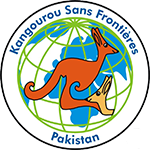### Unlimited Printable Worksheets, Online Practice and Online Tests For Kangaroo-Cadet• The duration of the competition is 3 hours.
• 30 problems to solve: ten – 3 points each, ten – 4 points each and ten – 5 points each.
• Calculators are not allowed. You may use basic geometric tools.
• Scope of problems:
• operations on rational numbers.
• powers of natural numbers.
• equations, inequalities and systems of linear equations.
• area of a rectangle, a triangle and a circle.
• lines and rays on a surface.
• volume and surface area of geometric figures.
• angles: acute, right, and obtuse.
• supplementary angles, sum of angles in a triangle and in a quadrilateral.
• mathematical logic
(Source : http://ikmc.kangaroo.org.pk/ )
Algebra, Integers, Symmetry, Data Handling, Logical Reasoning, Fractions, Mensuration - Perimeter, Area, Volume, etc., Geometry, Exponents and Powers, Decimals, Rational Numbers, Percentages, Ratio & Proportions, . . .

Algebra, Rational Numbers, Square and Square Roots, Graphs, Cubes and Cube Root, Exponents and Powers, Mensuration - Perimeter, Area, Volume, etc., Data Handling, Unitary Method, Percentages, Logical Reasoning, . . .